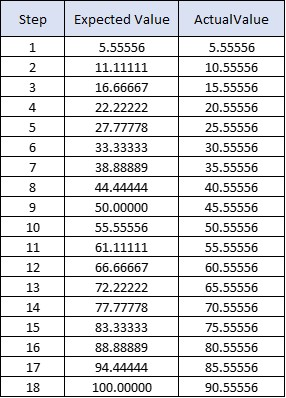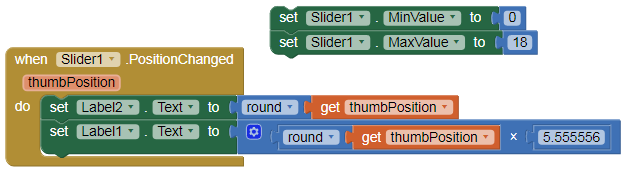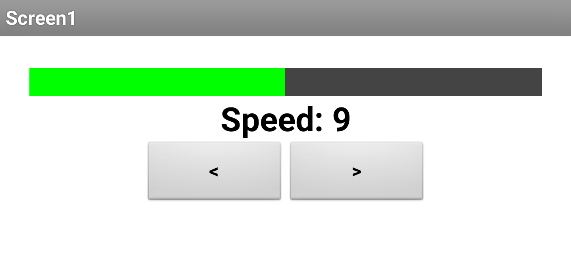# Math is truncating decimals

The app is truncation decimals.

The first time I trigger the function it correctly display 5.5556

When I add 5.5556 to that, it displays 10.5556. Adding 5.5556 to that gives 15.5556.

Does the slider thumb position value get truncated automatically?

Where is the truncation ? Am I missing something ?

Oh, I see it now…Looks like, at best, the slider will only return 2 decimal places, if setup correctly.

It’s displaying 5 decimals places in the text box.

It’s the unexplained truncation that’s puzzling.

What are the min / max values of the slider?

Note: The slider can display a maximum of 5 decimal places:

Anke,

I have been searching but do not know how to activate “Do it”.

The Min is 0 (Zero) and the Max is 100.

I am trying to get the Slider to advance in 5.556 increments so that 18 advances gets it from 0 to 100.

To provide context, the slider is being used as an indicator of the % of power being given to a motor. When a speed button is pressed it increases the speed one increment of 1/18th.1 Like

Connect to Companion, right mouse click: “Do it”
see here:

In this case, SliderThumbPosition returns only integer values.
If the min value = 0 and max = 10, it returns 1 decimal place.
Min = 0 and max = 1 it returns 2 decimal places, and so on.

1 Like

To make it short: The slider distributes 100 to the respective interval [0, 100], [0, 10], [0, 1] and seems to be therefore rather unsuitable for your purposes.

1 Like

A List Picker would take up less screen space and give you exactly the set of values you want.

1 Like

Yes, I agree.

On the bright side, I did get the Do It to work. That is a helpful tool.

1 Like

APG,

Thanks for the reply. I am not looking to pick the number. The context is the slider is being used as an indicator of the of power (speed) being given to a motor for a model train. When a arrow button is pressed it increases the speed one increment of 1/18th.

The slider (Green and Gray) would increase or decrease as the left and right arrows are pressed.

In your situation, I would make my own slider with a canvas and a ball for the thumb. You can even make a vertical slider in this way too.

1 Like@Juan_Antonio Sorry Juan, but what do we learn from this?

I don’t know if this is what they are looking for …. this code creates the column of the table “Expected value” of post 8.

1 Like

I am using the Slider as an Output, not an Input.

You click the right arrow (accelerate) and 1/18 is added to the current value of the slider.

The trouble is it behaves like this.
Slider set = 0.
Slider = 0, click the arrow, add 5.5556 to the slider. Slider = 5.55556. (0 + 5.5556)
Click the arrow again, add 5.5556 to the slider, Slider now = 10.5556 when it should be 11.1112.

I tried working with 1 to 10000.

It adds 55.556 and 55.556 and gives 105.556

I did some “Do Its” and saw that for some reason the system changes the value to 50 before it adds the next 55.556. That explains the numbers but not why it does that. Same thing with 1 to 100. It changes 5.5556 to 5.0 before the addition.

I’ll keep fiddling with it.

1 Like

Here is a way to do it just using a horizontal arrangement and a label:

outputslider.aia (3.2 KB)1 Like